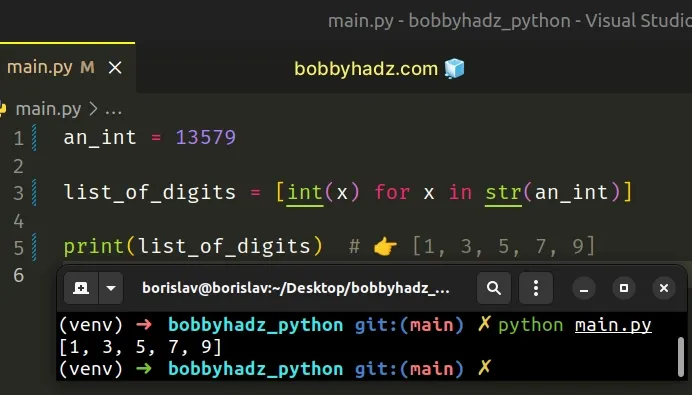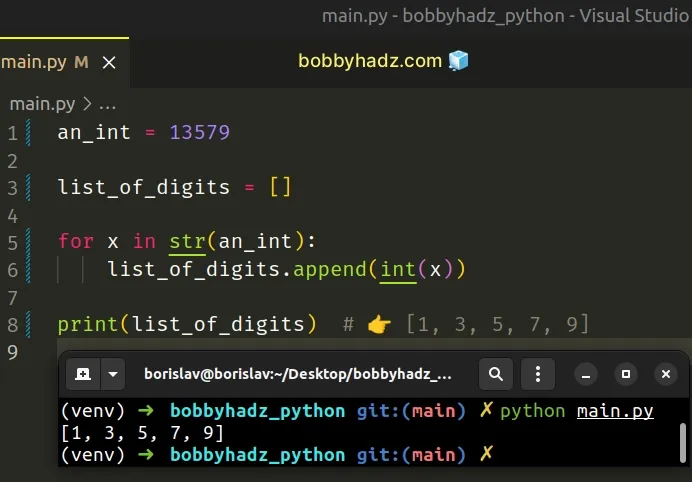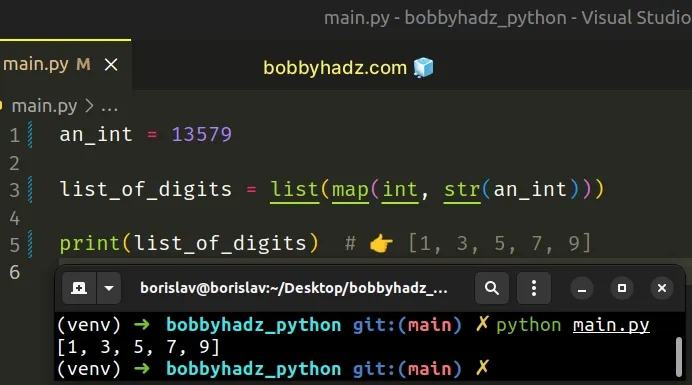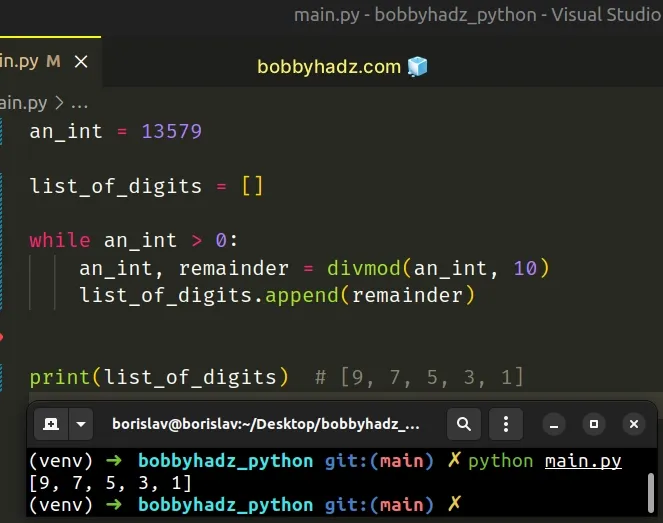# How to Split an Integer into Digits in PythonLast updated: Feb 19, 2023
4 min## #Split an integer into digits in Python

To split an integer into digits:

1. Use the `str()` class to convert the integer to a string.
2. Use a list comprehension to iterate over the string.
3. On each iteration, use the `int()` class to convert each substring to an integer.
main.py
```Copied!```an_int = 13579

list_of_digits = [int(x) for x in str(an_int)]

print(list_of_digits)  # 👉️ [1, 3, 5, 7, 9]
``````We used the `str()` class to convert the integer to a string, so we can iterate over the string.

The next step is to use a list comprehension to iterate over the string.

List comprehensions are used to perform some operation for every element, or select a subset of elements that meet a condition.

On each iteration, we pass the string to the int() class to convert it to an integer.

You can also use a simple for loop to achieve the same result.

## #Split an integer into digits using a `for` loop

This is a three-step process:

1. Use the `str()` class to convert the integer to a string.
2. Use a `for` loop to iterate over the string.
3. Use the `int()` class to convert each substring to an integer and append them to a list.
main.py
```Copied!```an_int = 13579

list_of_digits = []

for x in str(an_int):
list_of_digits.append(int(x))

print(list_of_digits)  # 👉️ [1, 3, 5, 7, 9]
``````We iterate over the digits that are wrapped in a string and on each iteration, we use the `int()` class to convert the value to an integer before appending the result to a list.

Alternatively, you can use the `map()` function to split an integer into digits.

## #Split an integer into digits using `map()`

This is a three-step process:

1. Use the `str()` class to convert the integer to a string.
2. Pass the `int` class and the string to the `map()` function.
3. Use the `list()` class to convert the `map` object to a list.
main.py
```Copied!```an_int = 13579

list_of_digits = list(map(int, str(an_int)))

print(list_of_digits)  # 👉️ [1, 3, 5, 7, 9]
``````The map() function takes a function and an iterable as arguments and calls the function with each item of the iterable.

Strings are iterable and integers are not, so the first step is to convert the integer to a string.
main.py
```Copied!```an_int = 13579

a_str = str(an_int)

list_of_digits = list(map(int, a_str))

print(list_of_digits)  # 👉️ [1, 3, 5, 7, 9]
``````

The `int()` class gets passed each substring from the string and converts the values to integers.

Note that the `map()` function returns a `map` object (not a list), so we have to use the `list()` class to convert the map object to a list.

## #Split an integer into digits using `math.ceil()` and `math.log()`

You can also use the `math.ceil()` and `math.log()` methods if you need to split the integer into digits without converting to a string.

main.py
```Copied!```import math

an_int = 13579

x = math.log(an_int, 10)
y = math.ceil(x)

list_of_digits = [(an_int//(10**i)) % 10 for i in range(y, -1, -1)
][bool(math.log(an_int, 10) % 1):]

print(list_of_digits)  # [1, 3, 5, 7, 9]
``````

The math.ceil method returns the smallest integer greater than or equal to the provided number.

main.py
```Copied!```import math

result_1 = math.ceil(25 / 4)
print(result_1)  # 👉️ 7

result_2 = 25 / 4
print(result_2)  # 👉️ 6.25
``````

The `math.log()` method returns the natural logarithm of a number.

You can also extract the logic into a reusable function.

main.py
```Copied!```import math

def split_integer(an_int):
x = math.log(an_int, 10)
y = math.ceil(x)

list_of_digits = [(an_int//(10**i)) % 10 for i in range(y, -1, -1)
][bool(math.log(an_int, 10) % 1):]

return list_of_digits

print(split_integer(12345)) # [1, 2, 3, 4, 5]
print(split_integer(100)) # [1, 0, 0]
print(split_integer(563)) # [5, 6, 3]
``````

The split integer function takes an integer as a parameter and splits the integer into a list of digits.

The solution is quite difficult to read, however, it is a bit faster because it doesn't require us to convert the value to a string.

## #Split an integer into digits using `divmod`

If you need to split an integer into digits from right to left, use the `divmod` method.

main.py
```Copied!```an_int = 13579

list_of_digits = []

while an_int > 0:
an_int, remainder = divmod(an_int, 10)
list_of_digits.append(remainder)

print(list_of_digits) # [9, 7, 5, 3, 1]
``````Notice that the result is produced from right to left.

The divmod function takes two numbers and returns a tuple containing 2 values:

1. The result of dividing the first argument by the second.
2. The remainder of dividing the first argument by the second.

On each iteration of the `while` loop, we use the `divmod()` function to get the result and the remainder of the division.

The remainder gets pushed into the list until the integer is equal to or less than `0`.

I've also written an article on how to split a float into integer and decimal parts.